# Understanding Probability in Pandemic Legacy: Season 2 – Part 2

Understanding Probability in Pandemic Legacy: Season 2 is a multi-part series. Links to the other parts can be found here:

Understanding Probability in Pandemic Legacy: Season 2 – Part 1
Probability of Incidents During Set-Up

Understanding Probability in Pandemic Legacy: Season 2 – Part 2
Probability of Incidents During Set-Up and How-To Use the Front 9 Calculator

Understanding Probability in Pandemic Legacy: Season 2 – Part 3
Strategies to Survive Between Set-Up and First Epidemic

Using the Front 9 Calculator

Use this calculator to help determine the best way to distribute supply cubes onto your game board prior to the Infection step of Set-Up.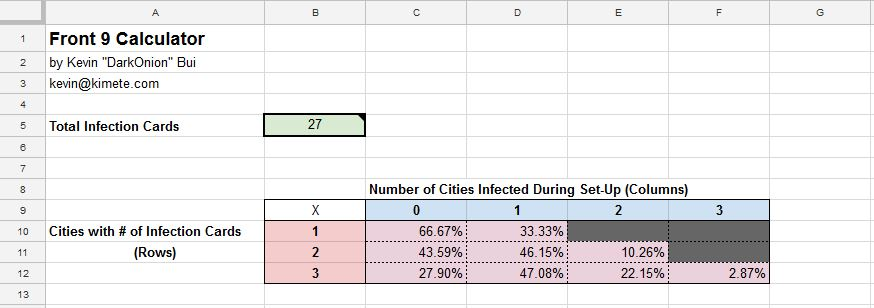In Part 1, we calculated the probabilities of drawing 0, 1, 2, or 3 cards of a city (Ex. New York) during the Infection step of Set-Up. In Google Sheets, using the built-in combination function [=combin(n, r)], we are easily able to fill out a table comparing the probabilities of Cities with # of Infection Cards vs. Number of Times a City Infected During Set-Up.

The calculator is provided here. It is write-protected so it won’t provide any spoilers of the game outside of the prologue. You will need to copy it to your own Google Drive to be able to edit it and use it.

The only cell you need to populate is B5 (cell filled green). As your Infection Deck grows or shrinks, update this cell to see the updated probabilities of an event happening.

Understanding the Values in the Front 9 Calculator

The vertical axis (the area shaded in red) represents the cities with 1, 2, or 3 city cards in the infection deck.

The horizontal axis (the shaded in blue) represents the number of times a city is infected during set-up (0, 1, 2, or 3).

The purple area represents probabilities of possible outcomes.

The gray area represents impossible outcomes (0% probability).

The sum of the outcomes of Row 1, Row 2, and Row 3 are 1 (or 100%). This is important as we will used this piece of information to work backwards and to derive context.

Examples

Example 1: What does Cell C11 represent? Infection Deck = 27. The city we are trying to calculate the probability for has only 2 cards in the Infection Deck. What is the probability that we will not draw any infection cards in this city during the Set-Up Infection?

= P(X = 0) or 1 – P(X > 0) or 1 – P(X = 1, 2, or 3)

= C11 = 1 – (D11 + E11 + F11) = 1 – (D11 + E11 + F11)

= 43.59%

In the context of Pandemic Legacy: Season 2: If no supply cubes where placed in this city, what’s the likelihood that this city will have an incident (Infection when no supply cues are present)?

Since there are no supply cubes, both outcomes of P(X = 1) and P(X =2) will cause an incident. Therefore the probability of an incident happening is:

P(X = incident when no supply cubes are present) =  P(X = 1) + P(X = 2)

= 0.4615 + 0.1026 = 0.5641

Example 2: What do Cells C12 to F12 represent? Infection Deck = 27. The city we are trying to calculate the probability for has only 3 cards in the Infection Deck. If we place a single supply cube on this city, what is the probability that we will not have any incidents during the Set-Up Infection?

Since there is a single supply cube in the city, the only way for the city to have an incident is if two or more infection cards are revealed during set-up. There are no incident outcomes if 0 or 1 infection card for this city comes up as the single supply cube negates the effect of the 1 infection card scenario.

= P(X = incident when a city with 3 cards in the infection deck has 1 supply cube on it)

Since we know that X = 0 and X = 1 will not cause an incident; and X > 1 (X = 2 and X = 3) will cause incident, the probability of an incident happening is:

= P(X = 2) + P(X = 3) = E12 + F12

= 0.2215 + 0.0287 = 0.2502

Theory Behind the Distribution Guidelines

Guidelines

1. Place one supply cube in each city that has 3 cards in the infection deck.
2. Place one supply cube in each city that has 2 cards in the infection deck.
3. Place one supply cube in each city that has 1 card in the infection deck.
4. Place a second supply cube in each city that has 3 cards in the infection deck.
5. Place a second supply cube in each city that has 2 cards in the infection deck.
6. Place a third supply cube in each city that has 3 cards in the infection deck.

Understanding the Numbers Behind the Guidelines

We already know this fact: The chance of getting an infection card from a city with 3 cards in the Infection Deck is higher than that of cities with only 2 cards in the Infection Deck. We also know that the probability that a city will be infected is higher for cities with two cards than it is for 1 cards.

Example 3:  There are no available cubes to distribute during set-up. List in order the type of cities (1 card, 2 cards, or 3 cards) that are most-likely to get at least one incident.

This information can be drawn from column C in the Front 9 Calculator. If we put context to these three cells, we would get this:

The probability that a city with 1 card in the infection doesn’t get an incident is 66.67%.
The probability that a city with 2 cards in the infection doesn’t get an incident is 43.59%.
The probability that a city with 3 cards in the infection doesn’t get an incident is 27.90%.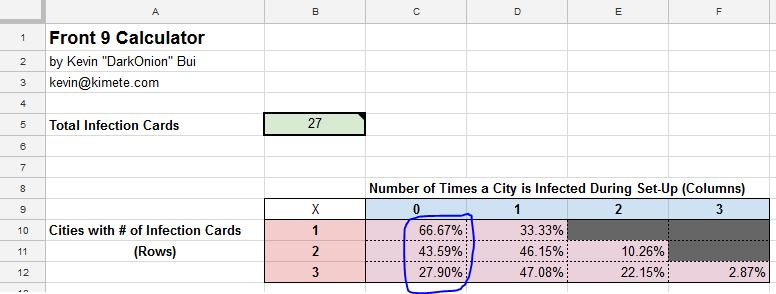Probabilities that cities with 1, 2, or 3 infection cards in the deck will not be infected.

Most Probable to Have at least One Incident
Cities with 3 Infection Cards in the Infection Deck
Cities with 2 Infection Cards in the Infection Deck
Cities with 1 Infection Card in the Infection Deck
Least Probable

This order of most to least probable will stay true as long the three types of cities have the same number of supply cubes on it. (Except when the number of supply cubes is greater or equal than the number of card cards in the Infection Deck. In that case, the outcomes has zero probability.)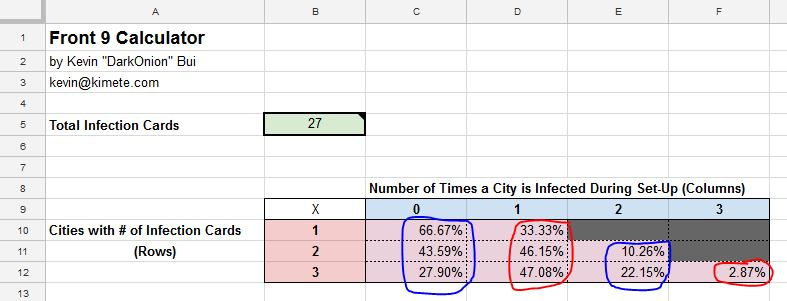The city with more infection cards in the Infection Deck will have a greater probability if all things else are equal.

Example 5: Place a supply cube in each of the cities that have 3 cities card in the Infection Deck. List in order the type of cities (1 card, 2 cards, or 3 cards) that are most-likely to get at least one incident.

We’ve already determine the probability of this scenario in Example 2 and know the probability is the sum of the outcomes where X > 1 (or cells E12 and F12).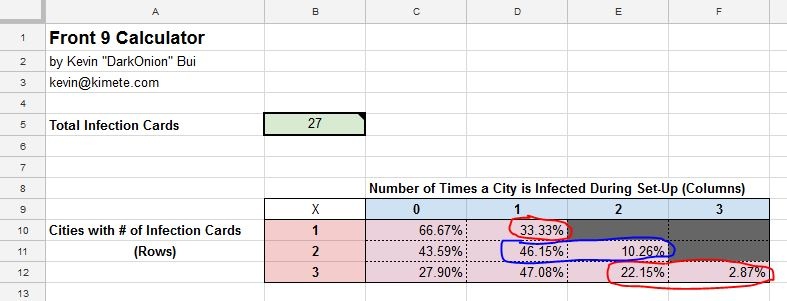The probability of an incident in a city with one supply cube in it and has 3 cards in the Infection Deck is greater less than the probability of an incident in a city with no supply cubes that has 1 or 2 cards in the Infection Deck.

Most Probable to Have at least One Incident
Cities with 2 cards and has 0 supply cubes. (Cells D11 + E11) = 0.5641
Cities with 1 cards and has 0 supply cubes. (Cell D10) = 0.3333
Cities with 3 cards and has 1 supply cube. (Cell E12 and F12) = 0.2502
Least Probable

Knowing these things, we are now able to order all the scenarios from most to least probable.

Most to Least Probable of All Scenarios

Most Probable to Have at least One Incident
Cities with 3 cards and 0 supply cubes. (Cells D12/E12/F12) = 0.7210
Cities with 2 cards and 0 supply cubes (Cells D11/E11) = 0.5641
Cities with 1 cards and has 0 supply cubes. (Cell D10) = 0.3333
Cities with 3 cards and has 1 supply cube. (Cells E12/F12) = 0.2502
Cities with 2 cards and has 1 supply cubes. (Cells E11) = 0.1026
Cities with 3 cards  and has 2 supply cubes. (Cell F12) = 0.0287
Cities with 1 cards in the Infection Deck and has 1 supply cubes. = 0
Cities with 2 cards in the Infection Deck and has 2 supply cubes. = 0
Cities with 3 cards in the Infection Deck and has 3 supply cubes. = 0
Least Probable

Distributing the Supply Cubes

Using the information above, we can now determine how to best minimize the chance of incidents. In ideal situations where you have unlimited supply cubes, you would just distribute 3 supply cubes to each 3 card city, 2 supply cubes to each 2 card city, and a single supply cube to each 1 card city for a 0% percent chance of incidents.

However, the real-game scenario limits the number of supply cubes that are available during Set-Up. In-game, you would want to prioritize the cities with the highest probability first. As you add cubes one by one to a city, the probability of incidents occurring will decrease.

Cities with 3 cards and 0 supply cubes has the highest probability of incidents. Once  a supply cube is added to the city, the probability of an incident decreases to 0.2502.

The strategy is to address the highest probability incident scenarios, not cities, first. Once that scenario is completely addressed, the next step is address the next highest probable scenario, cities with 2 cards and 0 supply cubes (0.5641). And so on.

When the allotted supply cubes are distributed with the above priority, the board state will have the least probable set-up for incidents. Of course, this is just by the numbers. Higher probability will happen more often. Lower probability will happen less often.

If this method doesn’t work for you, my is disclaimer is this: This method doesn’t take into account luck, misfortune, poor shuffling, and/or Acts of God.

# Understanding Probability in Pandemic Legacy: Season 2 – Part 1

Oh my god. I am actually using my degree.

Before I begin, I just wanted everyone to know that I am going to try my best to not spoil any of the game other than what you might have seen in the Prologue month.

Understanding Probability in Pandemic Legacy: Season 2 is a multi-part series. Links to the other parts can be found here:

Understanding Probability in Pandemic Legacy: Season 2 – Part 1
Probability of Incidents During Set-Up

Understanding Probability in Pandemic Legacy: Season 2 – Part 2
Probability of Incidents During Set-Up and How-To Use the Front 9 Calculator

Understanding Probability in Pandemic Legacy: Season 2 – Part 3
Strategies to Survive Between Set-Up and First Epidemic

Understanding Probability in Pandemic Legacy: Season 2

Pandemic Legacy: Season 2

Game play of the Prologue month can easily be found on YouTube and the month is often used to introduce the game play, mechanics, and work flow to new players. The prologue has no permanent effects on the game and can be played an unlimited number of times before starting the January month.

For those of you who are in a current game of Pandemic Legacy: Season 2, you might have noticed that the game is quite a bit harder than Season 1. You aren’t alone. It seems like everyone is struggling to meet their monthly objectives. Here are some tips.

1. Review the contents of the Infection Deck. Count the number of cards and see how the city infection cards are distributed. At the beginning of the game, there are 27 cards in the infection deck. 9 cities with 3 card each. This will change as the game progresses. As you are playing the game, keep track of which cards came up. This will help you determine the likelihood of future cards.After each epidemic, before shuffling the infection deck discard and putting it on top of the infection deck, review the played cards and take notes. Again, this will help you prepare for the upcoming infections.
2. Review the contents of the player deck. Most importantly, get an idea of how often an Epidemic will come up. If the player deck has 60 cards, and there are only 5 Epidemics in the game, you should expect an Epidemic in each 12 card cycle.
3. Take Notes. Tally the number of players cards that you have gone through. Tally the number of cities that have come up. Tally how many turns/player cards since the last epidemic, or which epidemic cycle you are currently on.

Solving for the Probability of Event (blank)

Now onto the probability. Why is probability so important? A core mechanic of Season 2 is keeping enough supply (gray) cubes in each city on the board to adequately protect the city from incidents, or infection of a city when there are no stockpile cubes left. When a city gets infected, a supply cube is removed. When a city is infected and has no supply cubes left, a disease cube is placed in the city and the incident marker is moved forward. When the game reaches the 8th incident for the game, Pandemic has won and that attempt for that month is over.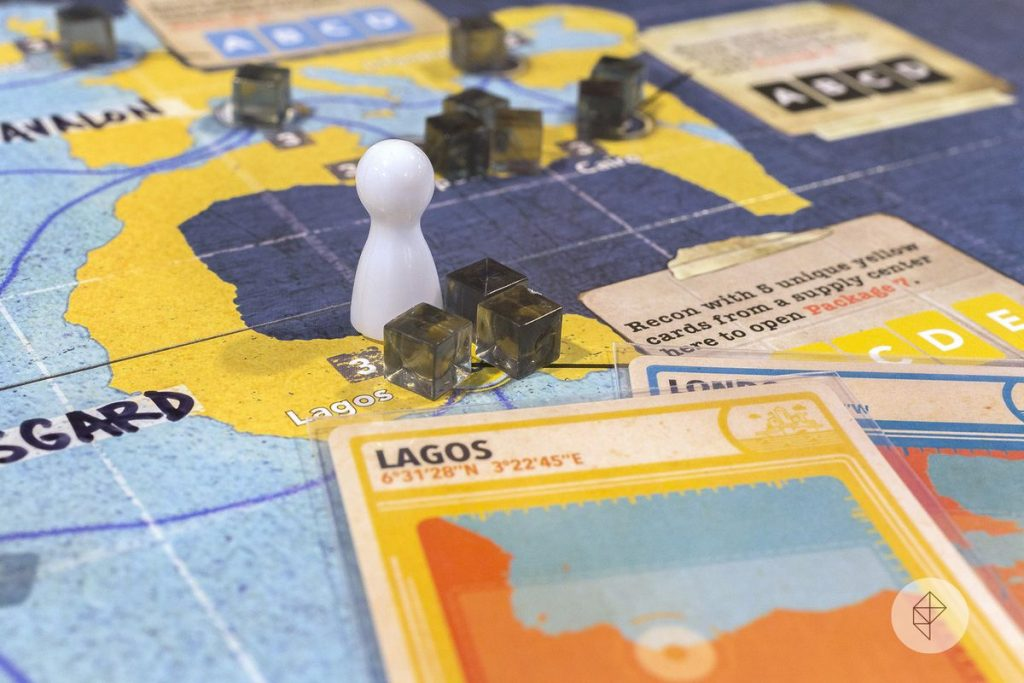Image Source: https://www.polygon.com/2017/8/24/16197080/pandemic-legacy-season-2-preview-release-date-gen-con

How do we determine how many stockpile cubes are adequate? How do we distribute stockpile cubes in such a way that we minimize the number of incidents occurring during the initial infection?

That’s really a great question. To best answer that, we need to make some assumptions as well as simplify the scenario a little bit.

Rules/Context

During the set-up of the game, the players have a limited number of supply cubes to distribute among the cities connected to the grid. For the prologue month, there’s 9 cities and 36 stockpile cubes that need to be distributed. The infection deck has 27 cards. 3 cards for each city connected to the grid. After the cubes are distributed, set-up does an initial infection by drawing 9 cards from the infection deck and infecting the 9 revealed cities.

Solution (Kind of.)

To answer the initial question, let’s simplify even more. Let’s only concentrate on a single city, New York. Of the 27 cards in the infection deck, 3 cards are New York. During the set-up infection (9 infection cards), what’s the likelihood that 0, 1, 2, or 3 New York cards show up? Does it make sense to place 0, 1, 2, or 3 cubes on New York to prevent incidents?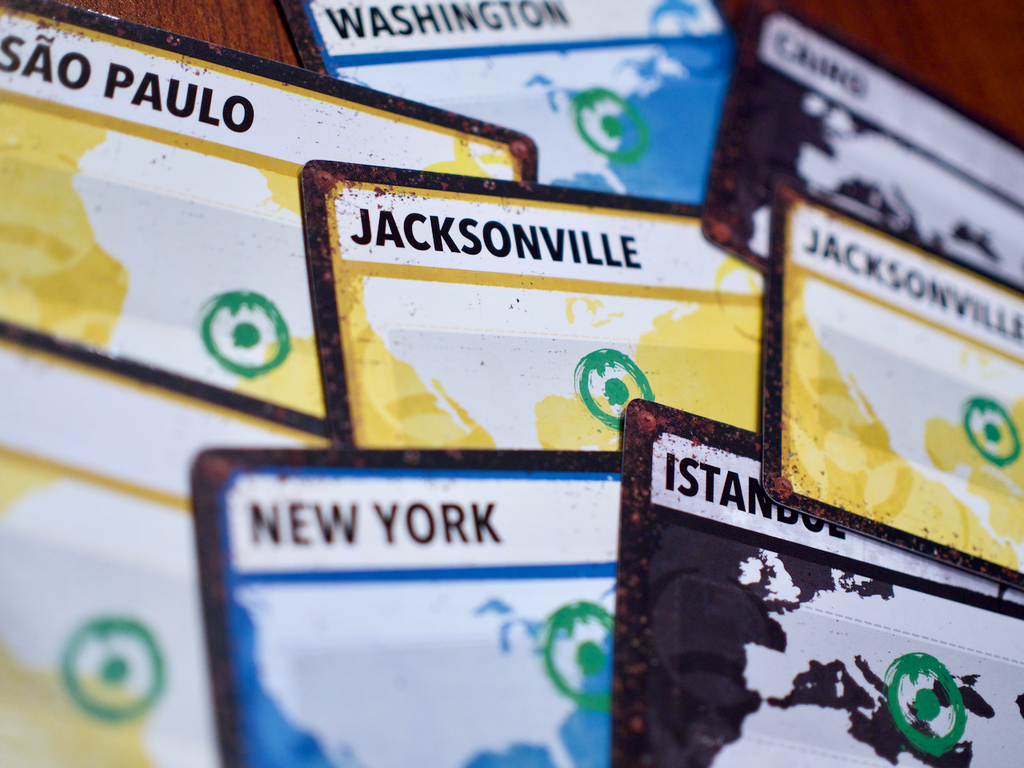Source: https://www.forthewincafe.com/blogs/news/ftw-staff-picks-pandemic-legacy-season-2

Defining some variables:

Deck size = N = 27
Total number of cards drawn = R = 9
Number of New York cards = n = 3
Number of New York Cards drawn = r = [0, 1, 2, or 3]

If we were only drawing one card, probability would be really easy to determine. P(X = Draw New York) = 3/27.

However, since we are drawing 9 infection cards, we have to factor in that New York cards can fall into any slots. We could draw a New York with the first card, or the last card, or any card in between. In order to account for this, we will use combinations as don’t care about order.

Some other assumptions: Though the remaining 24 cards in the Infection Deck are not all the same, for this problem, we’ll just lump them together and consider them “Non-New York.”

Reference:

https://betterexplained.com/articles/easy-permutations-and-combinations/

For reference:

Combinations = nCr = C(n, r)
Solve in Google Sheets using this function: =combin(n, r)

P(X = r) =

C(n, r) * C(N-n, R-r)
——————
C(N, R)

In layman’s terms,

C(n, r) is the number of ways to draw r New York card from n New York cards.

C(N, R) is the number of ways to draw R (R=9) cards, from N (N=27).

C(N-n, R-r) refers to the number of ways to draw R-r (Non-New York) from N-n (Non-New York)

P(X = Draw 1 New York)

P(X = Draw 1 New York) =

[Combination(of drawing 1 New York from 3 New York cards) x Combination(of drawing 8 Non New York cards from 24 Non-New York Cards)] / [Combination(of drawing 9 cards from 27)]

It should look something like this:

C(3,1) * C(24, 8)
——————
C(27, 9)

P(X = Draw 1 New York) = 0.4708

P(X = Draw 2 New York)

Let’s try for P(X = Draw 2 New York).

C(3,2) * C(24, 7)
——————
C(27, 9)

As you can see, only the numerator changes. C(3,2) refers to drawing 2 New York’s from a total of 3. While C(24,7) refers to the number of ways to draw the 7 remaining cards from the Non New York subset.

P(X = Draw 2 New York) = 0.2215

P(X = Draw 3 New York)

C(3,3) * C(24, 6)
——————
C(27, 9)

P(X = Draw 3 New York) = 0.0287

P(X = Draw 0 New York)

Just for due diligence, let’s also do P(does not draw New York).

C(3,0) * C(24, 9)
——————
C(27, 9)

P(X = 0 New York) = 0.2790

Results

P(X = 0) = 0.2790
P(X = 1) = 0.4708
P(X = 2) = 0.2215
P(X = 3) = 0.0287

Let’s interpret the results.

P(X = 0) = 0.2790 – Probability that New York gets exactly 0 infections
P(X = 1) = 0.4708 – Probability that New York gets exactly 1 infection
P(X = 2) = 0.2215 – Probability that New York gets exactly 2 infections
P(X = 3) = 0.0287 – Probability that New York gets exactly 3 infections

Scenario:

Ex. I put only supply cubes on New York. What’s the risk or likelihood that New York will have an incident during the initial infection set-up? (What’s the probability that New York will be infected 3 times during Set-Up?)

P(X > 2) = 1 – P(X > 2) = P(X = 3) = 0.0287

Ex. I put one supply cube in New York. What’s the risk or likelihood that New York will have an incident during the initial infection set-up? (What’s the probability that New York will be infected 2 or more times?)

P(X > 1) = P(X = 2, 3) = P(X = 2) + P(X = 3) = 0.2215 + 0.0287 = 0.2502

Other Scenarios

Ex. There are only two New York Infection cards in the deck. (2 New York cards and 25 Non-New York cards) What are the probabilities of P(X = 0), P(X = 1), and P(X = 2)?

Deck size = N = 27
Total number of cards drawn = R = 9
Number of New York cards = n = 2
Number of New York Cards drawn = r = [0, 1, or 2]

The set-up of the formula is the same except that we’ll be using the new numbers above to substitute them into the combinations formula.

For P(X = 0), the formula will look like this:

C(2,0) * C(25, 9)
——————
C(27, 9)

P(X = 0) = 0.4359

Furthermore: P(X = 1) = 0.4615 and P(X = 2) = 0.1026.

Set-Up Infection Calculator in Google Docs

I’ve set up this cool little table/calculator to determine the probability that cities will become infected with 0, 1, 2, or 3 infection cubes during set-up. Just update your Infection Deck size in the green box.

Pandemic Legacy: Season 2 – Front 9 CalculatorConclusion

What’s the best way to distribute supply cubes during set-up? Using the Set-Up Infection Calculator and the combination theories above, the best guidelines are to distribute supply cubes in this order. Continue to the next point if you still have stockpile cubes still available. Proof of this strategy can be found in Part 2.

1. Place one supply cube in each city that has 3 cards in the infection deck.

2. Place one supply cube in each city that has 2 cards in the infection deck.

3. Place one supply cube in each city that has 1 card in the infection deck.

4. Place a supply stockpile cube in each city that has 3 cards in the infection deck.

5. Place a supply stockpile cube in each city that has 2 cards in the infection deck.